# NLP教程(9) - 句法分析与树形递归神经网络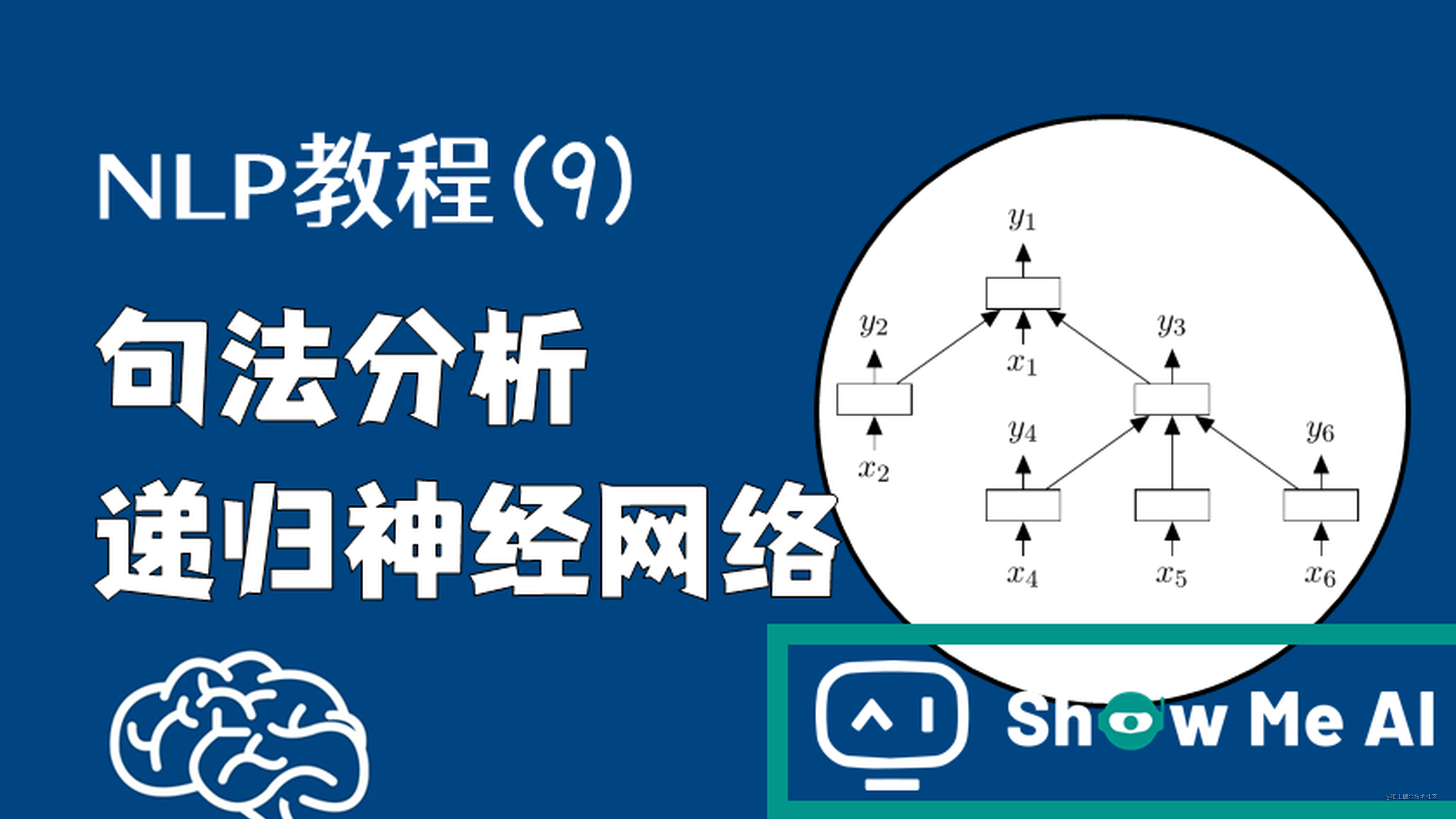ShowMeAI为CS224n课程的全部课件，做了中文翻译和注释，并制作成了 GIF动图！点击 第18讲-句法分析与树形递归神经网络 查看的课件注释与带学解读。更多资料获取方式见文末。

# 引言

CS224n是顶级院校斯坦福出品的深度学习与自然语言处理方向专业课程，核心内容覆盖RNN、LSTM、CNN、transformer、bert、问答、摘要、文本生成、语言模型、阅读理解等前沿内容。

## 笔记核心词

• 树形模型
• 递归神经网络 / Recursive Neural Networks
• 句法分析 / Constituency Parsing
• 成分句法分析
• SU-RNN
• MV-RNN
• RNTN

# 1.递归神经网络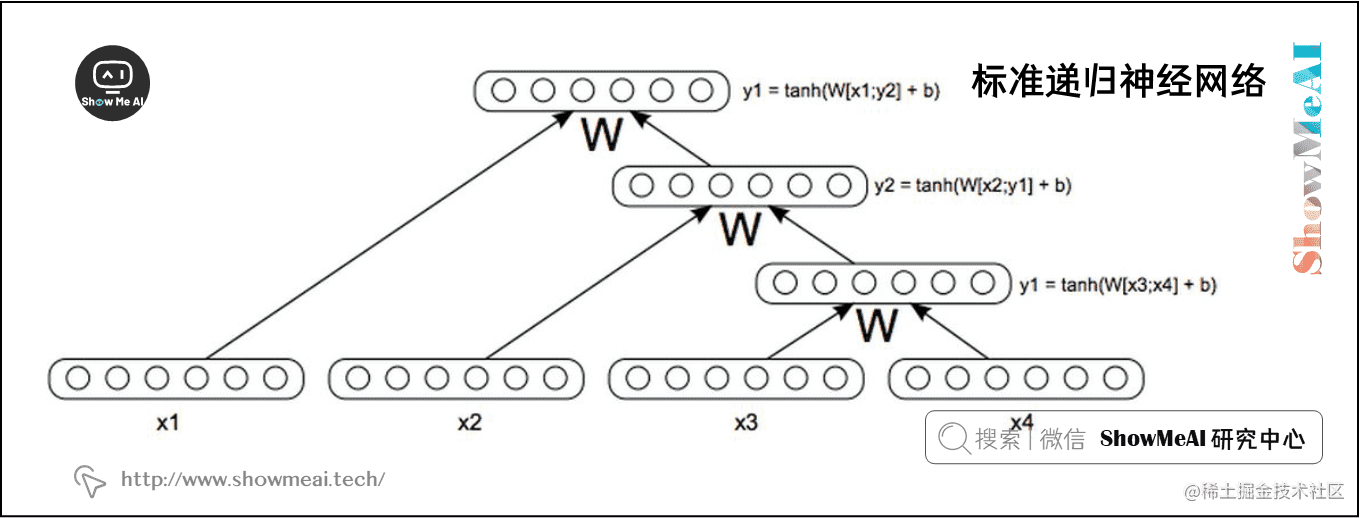1. 单词的组合有无数种可能。存储和训练无限数量的向量将是荒谬的。
1. 有些单词的组合虽然在语言中完全可以听到，但可能永远不会出现在我们的 训练 / 开发 语料库中，所以我们永远学不会。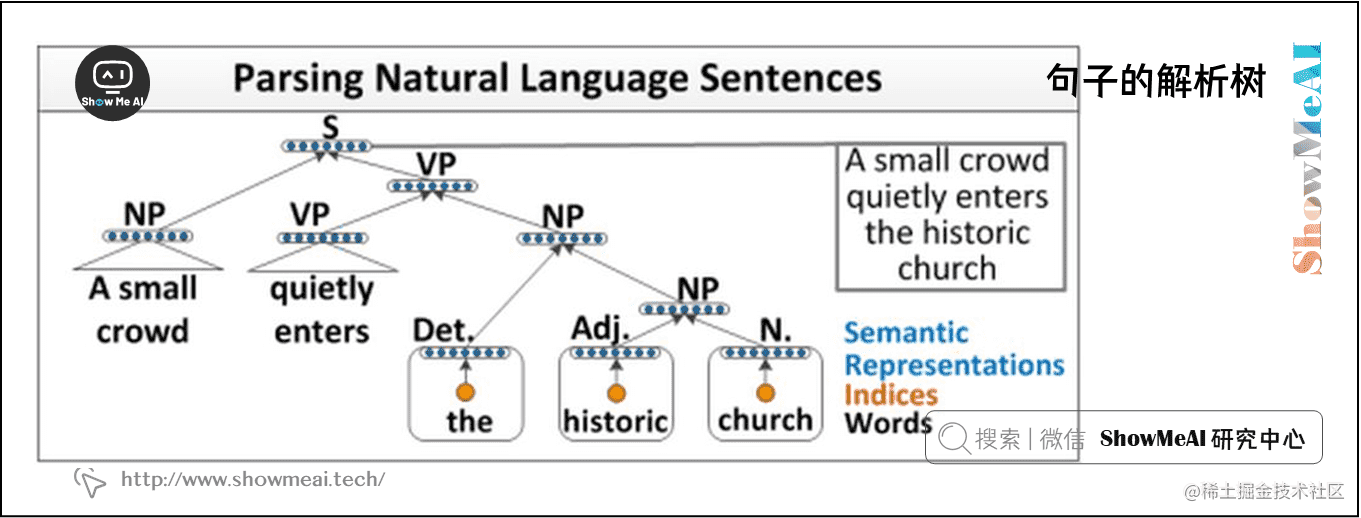## 1.1 递归神经网络的单层结构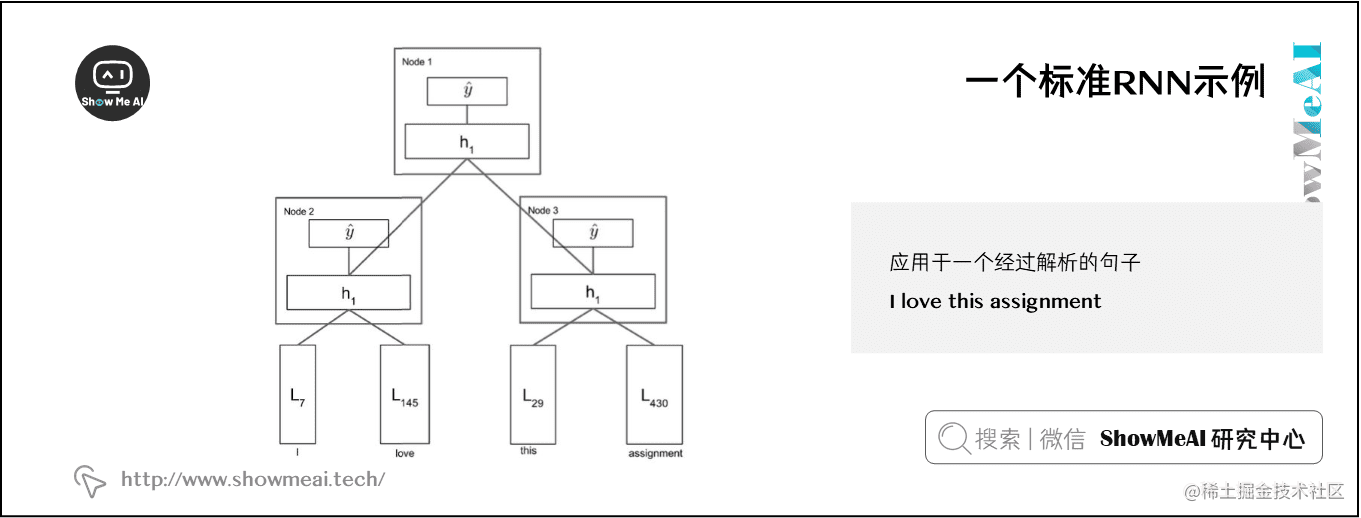$h^{(1)}=\tanh \left(W^{(1)}\left[\begin{array}{c}{L_{29}} \ {L_{430}}\end{array}\right]+b^{(1)}\right)$

$h^{(1)}=\tanh \left(W^{(1)}\left[\begin{array}{c}{h_{L e f t}^{(1)}} \ {h_{R i g h t}^{(1)}}\end{array}\right]+b^{(1)}\right)$

## 1.2 Syntactically Untied SU-RNN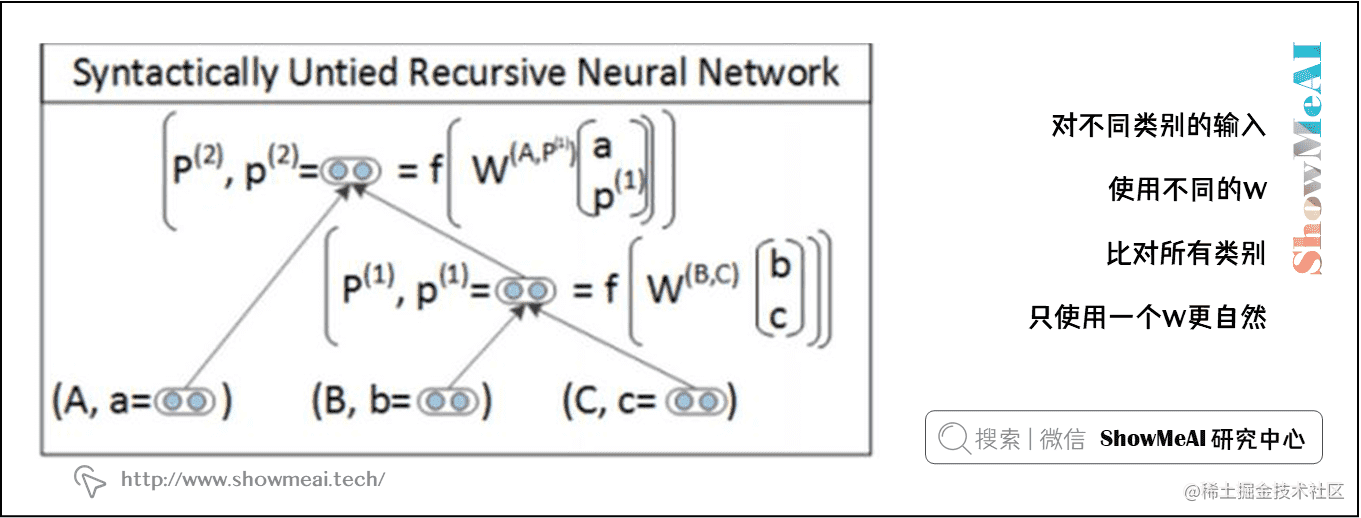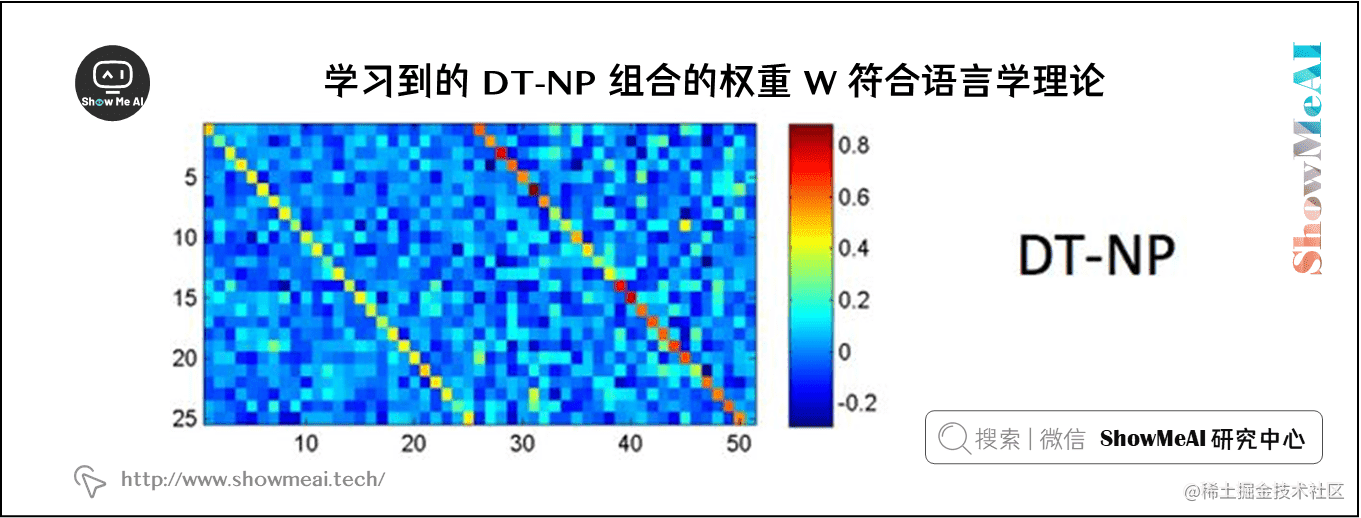SU-RNN确实比之前讨论过的模型表现得更好，但也许它的表现力还不够。如果我们考虑修饰词，比如副词 very ，任何与这个单词向量和下面这个单词向量的插值，肯定不是我们所理解的 very 的本质。

## 1.3 MV-RNN (Matrix-Vector Recursive Neural Networks)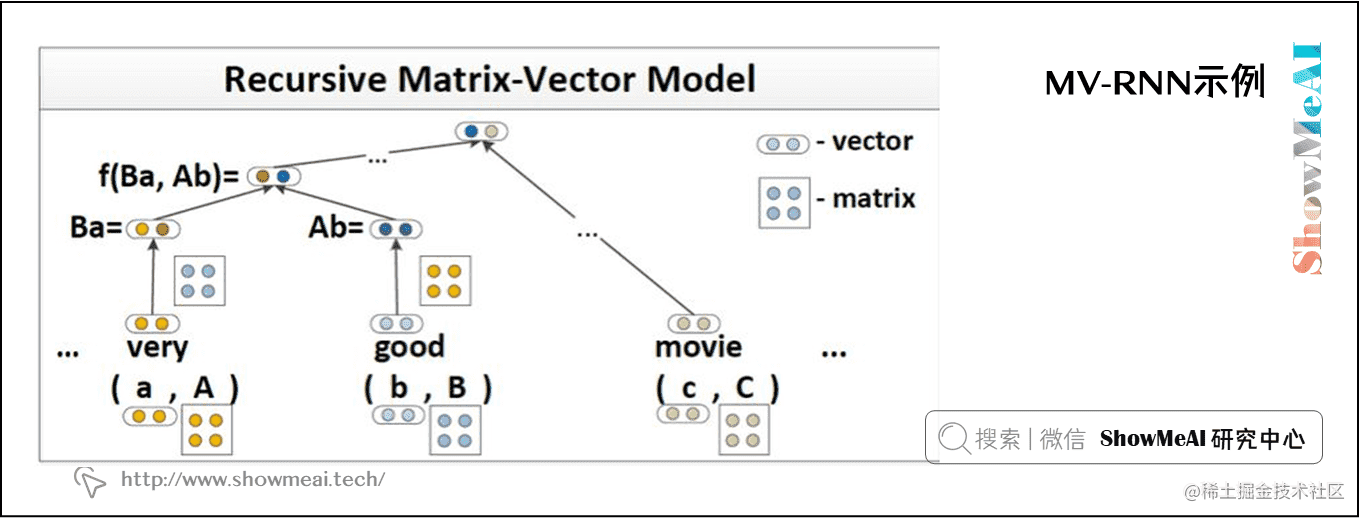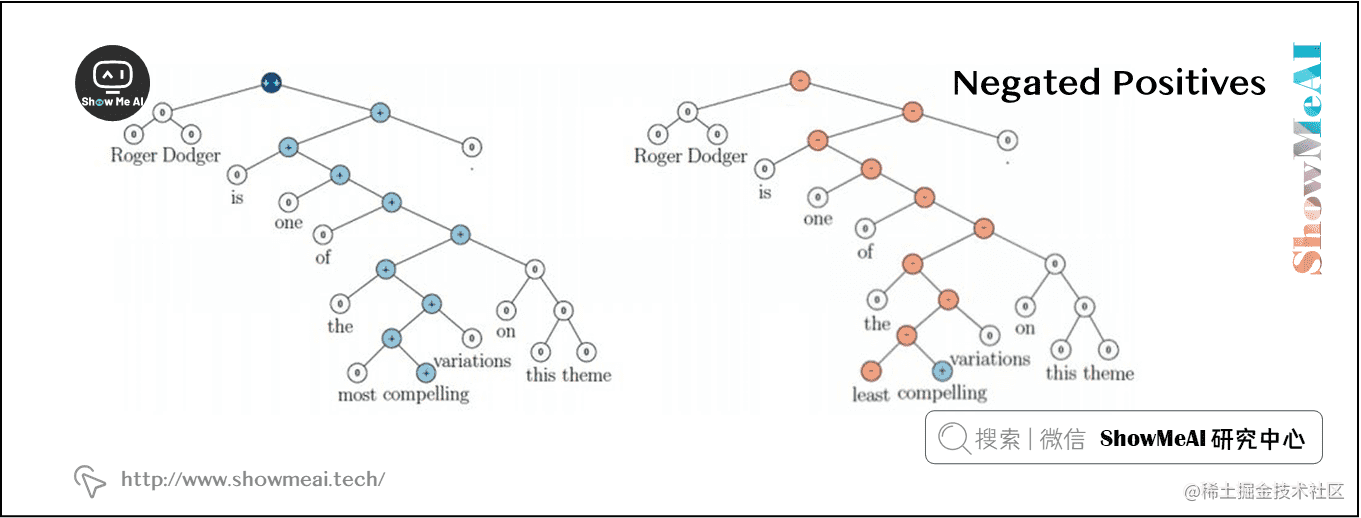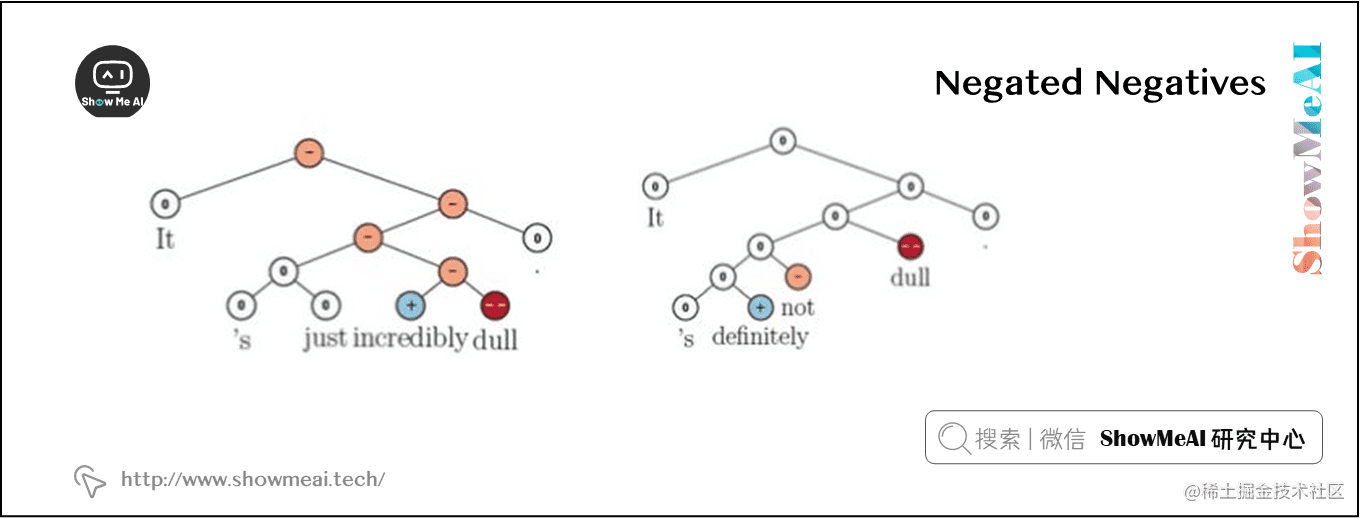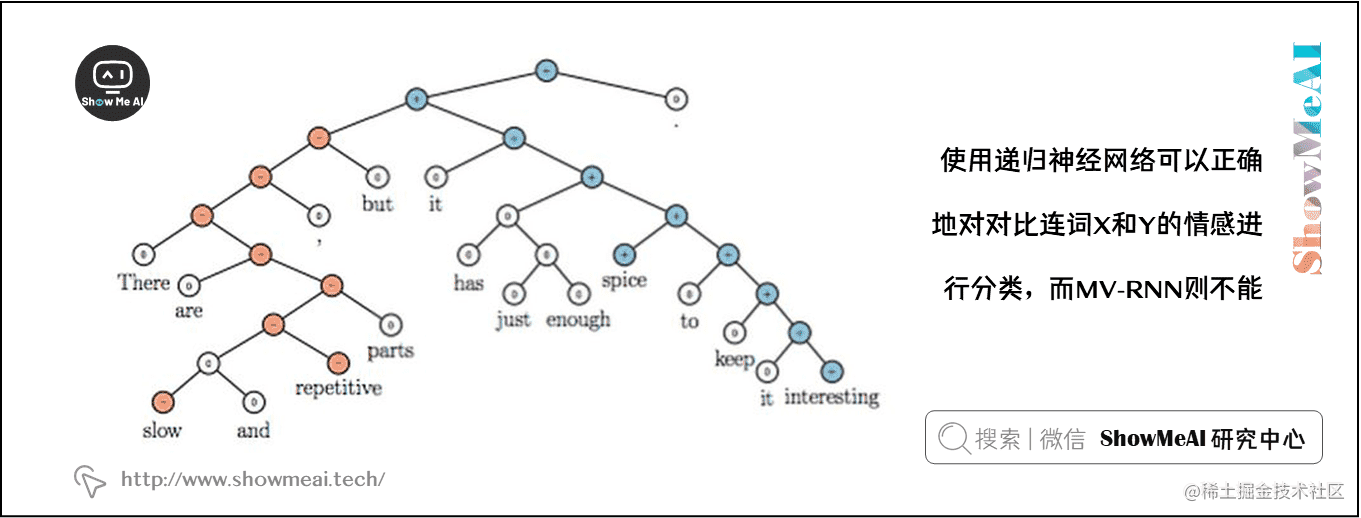## 1.4 RNTN (Recursive Neural Tensor Network)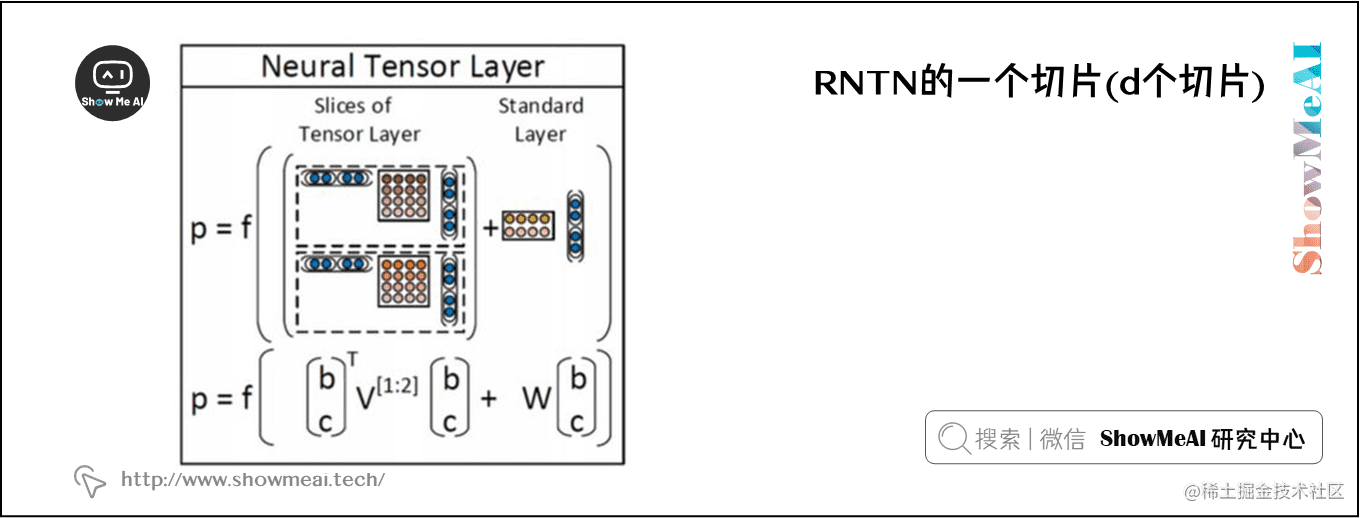$h^{(1)}=\tanh \left(x^{T} V x+W x\right)$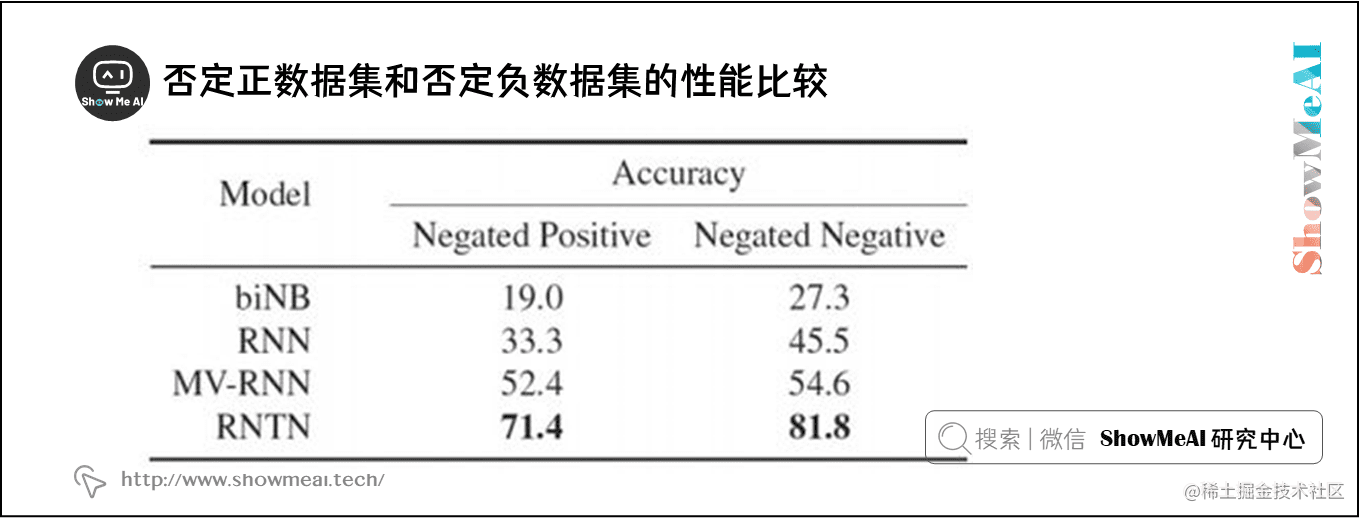# 2 成分句法分析

## 2.1 成分

• I want to be enrolled in the wonderful CS224N!

• The wonderful CS224N I want to be enrolled in

• I want to be enrolled in the great CS course in Stanford about NLP and Deep Learning!

• $\mathrm{S} \rightarrow \mathrm{NP} \quad \mathrm{VP}$

• Colorless green ideas sleep furiously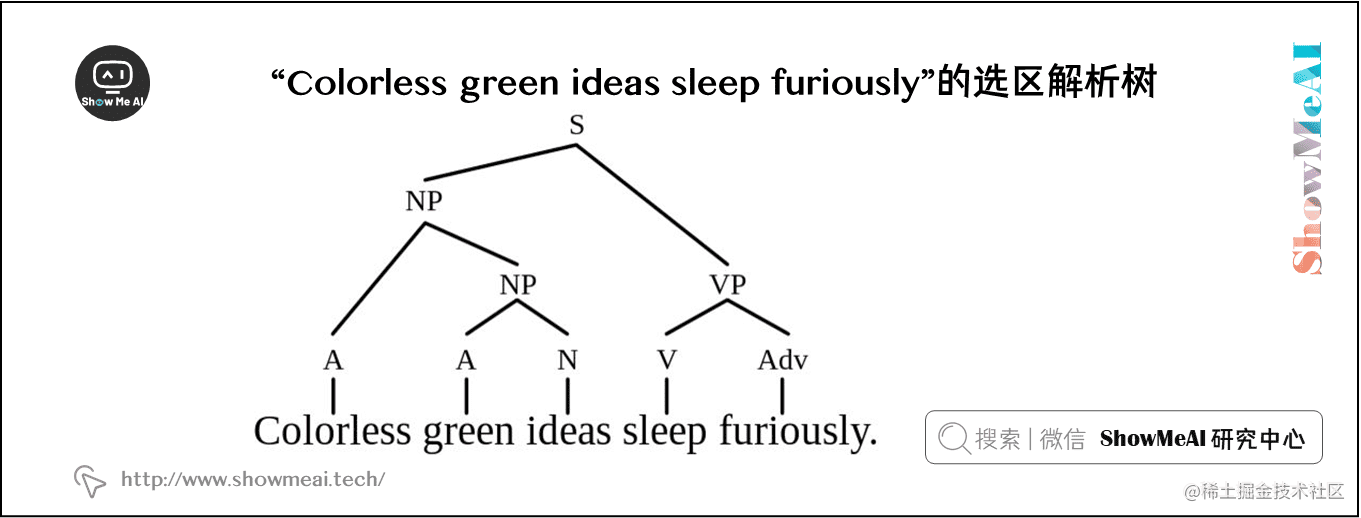## 2.2 成分句法解析树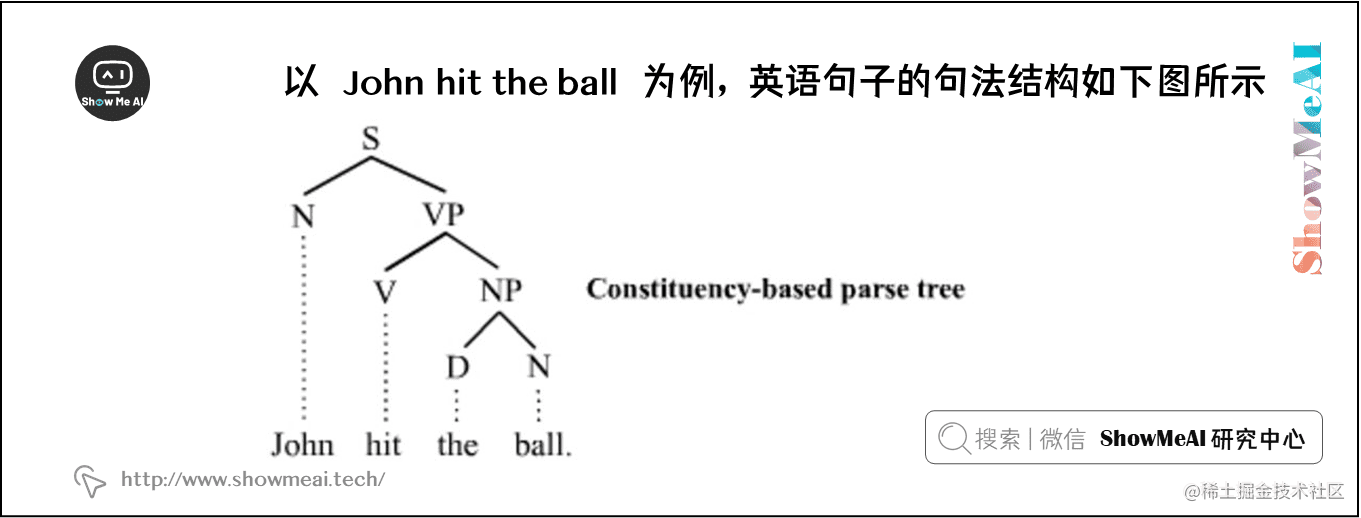• S 代表句子，最高级的结构
• NP 代表名词短语，包括句子的主语和宾语
• VP 代表动词短语，充当谓语
• V 代表动词
• D 代表限定词，例如 the
• N 代表名词

# 斯坦福 CS224n 课程带学详解